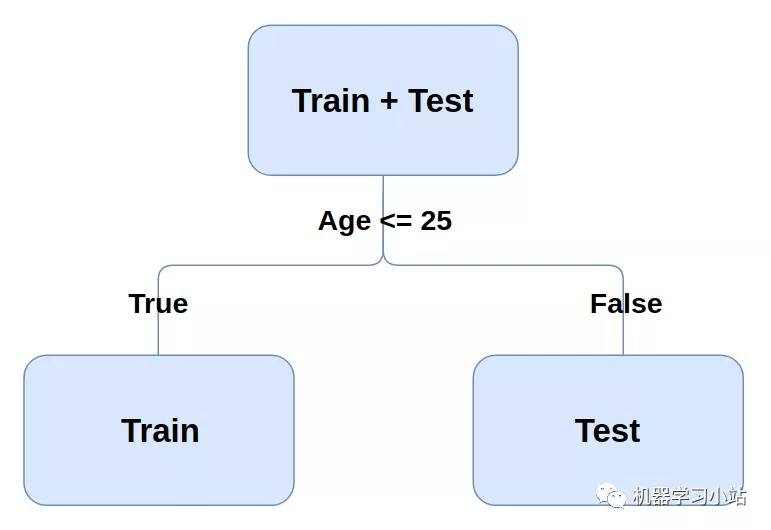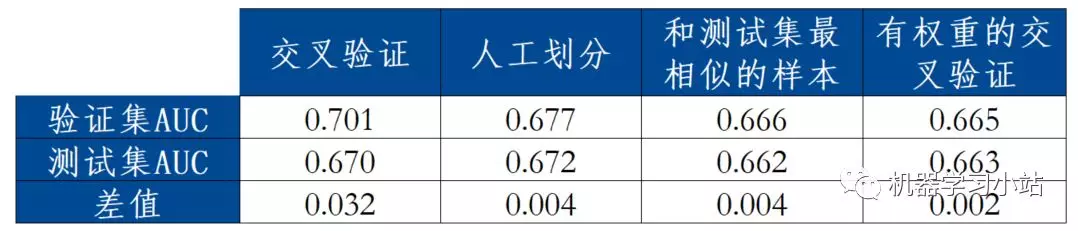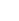# 目录

• 什么是样本分布变化
• 为什么样本分布变化的时候，交叉验证不适用？
• 什么是对抗验证？
• 分布变化时，有哪些优于交叉验证的方法？
• Kaggle比赛实例（lightgbm模型；Python）

# 2 为什么「样本分布变化」的时候，交叉验证不适用？1. 定义y：样本是train还是test。
2. 将 Train 和 Test 合成一个数据集
3. 构造一个模型，拟合新定义的y
4. 观察模型效果：如果模型的AUC超过0.7，说明了 Train 和 Test 的分布存在较大的差异
``````# 定义新的Y
df_train['Is_Test'] = 0
df_test['Is_Test'] = 1

# 将 Train 和 Test 合成一个数据集。
``````

# 4 分布变化时，有哪些优于交叉验证的方法？

## 4.1 人工划分验证集

``````# 将样本根据时间排序
df_train = df_train.sort_values('Date').reset_index(drop=True)
df_train.drop(['Date'], axis=1, inplace=True)

# 前80%的样本作为训练集，后20%的样本作为验证集
df_validation_1 = df_train.iloc[int(0.8 * len(df_train)):, ]
df_train_1 = df_train.iloc[:int(0.8 * len(df_train)), ]
``````

## 4.2 和测试集最相似的样本作为验证集

``````# 通过抗验证中的模型，得到各个样本属于测试集的概率

# 只需要训练样本的概率
df_train_copy = df_train.copy()

# 根据概率排序
df_train_copy = df_train_copy.sort_values('is_test_prob').reset_index(drop=True)

# 将概率最大的20%作为验证集
df_validation_2 = df_train_copy.iloc[int(0.8 * len(df_train)):, ]
df_train_2 = df_train_copy.iloc[:int(0.8 * len(df_train)), ]
``````

## 4.3 有权重的交叉验证

``````# 生成lightgbm的数据格式，赋予各个样本权重
train_set = lgb.Dataset(
df_train.drop('HasDetections', axis=1),
``````

# 5 实例

## 5.1 用到的数据## 5.2 对比各种方法的效果# 6 结论##### 相关文章客服诗玥：2261033423客服娜娜：1927588323客服紫薇：2302909291